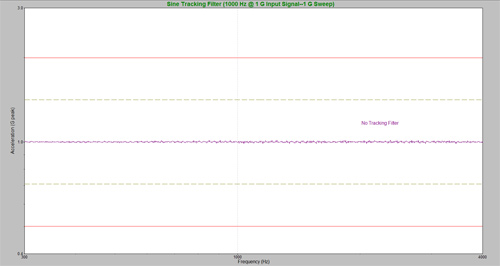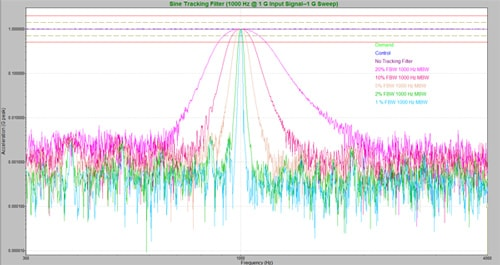Back to Articles

# Sine Tracking Filters to Remove Harmonics and Noise

#### Data Acquisition

When running a sine test, the main interest is the amplitude of the output frequency or the “pure tone.” Sine tracking filters isolate the pure sine tone generated by the drive output.

Test engineers want to make sure a product is tested with the specified amplitude at the specified frequency. Due to the inherent noise of a shaker system, however, a controller will pick up contributions from noise and harmonics in addition to the pure tone. The background noise alters the reading of the pure tone’s amplitude.

### Theoretical Example

Let’s run a sine sweep from 5Hz to 100Hz with a 1G amplitude. As the sweep advances, let’s look at a moment in time at 10Hz. The drive is outputting 1G but the input is acquiring noise from the other frequencies (5Hz, 20Hz, 50Hz, 100Hz, etc).

Perhaps there is a harmonic at 100Hz contributing 0.5G and system noise at 50Hz contributing 0.3G. The controller concludes there is 0.8G at 10Hz. It adjusts the drive output to account for the reading at 10Hz and only outputs the remaining 0.2G required to reach 1G at 10Hz.

The controller now measures a total of 1G at 10Hz. However, it is not outputting 1G at 10Hz but 0.2G at 10Hz. The amplitude of the output frequency has been adjusted significantly. The test is not producing desired results, as the main interest in a sine test is the amplitude of the output frequency.

### The Solution

A sine tracking filter is used to isolate the pure sine tone so the controller can obtain an accurate reading of the pure tone’s amplitude and accurately control the pure tone. It is called a tracking filter because it tracks along as the test moves through different frequencies, filters out noise at frequencies of non-interest, and keeps the bandwidth narrow, thereby isolating the pure sine tone at each frequency.

In the example above, amplitude readings at frequencies other than 10Hz were affecting the test. By applying the sine tracking filters to our test, the noise at 100Hz (0.5G) and 50Hz (0.3G) is filtered out. At the same moment in time at 10 Hz, the controller now measures 0G at 10Hz rather than 0.8G and adjusts the drive to output a full 1G at 10Hz. The test is now producing the desired results.

### Tracking Filters Experiment

The above example was a simple explanation to demonstrate how the frequencies of non-interest affect control readings. In reality, the vibration controller makes adjustments based on the average RMS of the defined bandwidth. The following experiment will provide a more in-depth explanation.

#### Setup

The VR9500 controller is set to run a sine sweep from 300Hz to 4,000Hz at 1G. The output is looped to the input. A 1,000Hz wave at 1G is generated by a function generator and fed into input Channel 2.

#### Without Tracking FiltersFigure 1. A 1G reading for all frequencies, even though there is a 0G output at all frequencies other than 1,000 Hz.

Without a tracking filter, the controller reads 1G over the entire bandwidth during the sweep. As a result, the controller plots 1G at every frequency. There is a 0G output at all frequencies other than 1,000 Hz, but the display shows a 1G reading for all these other frequencies. Why?

As the controller moves through every frequency, it considers the entire bandwidth and not just the frequency of interest. The bandwidth has a 1G acceleration at all times, but the individual frequencies are not contributing any acceleration to the bandwidth (except at 1,000Hz).

Without a tracking filter, the controller reads the acceleration from all frequencies and controls based on the average acceleration. The acceleration from noise and harmonics doesn’t belong to the frequency of interest but all unfiltered contributions are averaged into the control.

#### With Tracking Filters

In VibrationVIEW‘s Sine software, the user defines the bandwidth of the tracking filter. The tracking filter bandwidth is defined as both a fractional bandwidth and maximum bandwidth.

A fractional bandwidth sets the bandwidth of the tracking filter as a percentage of the output frequency, not the total bandwidth. For example, consider a 20% fractional bandwidth. When the sine sweep (output frequency) is at 10Hz, the tracking filter bandwidth is 20% of 10Hz or 2Hz. With a 2Hz tracking filter bandwidth and at a 10Hz output frequency, the control averages the accelerations from 9Hz to 11Hz and filters out all other frequencies.

The fractional bandwidth increases as the output frequency increases. For example, at 10Hz, a 20% fractional bandwidth is 2Hz. At 2,000Hz, however, the fractional bandwidth is 400Hz. At this frequency, noise from anywhere between 1,800Hz and 2,200Hz could contribute to the reading.

The maximum bandwidth sets the maximum allowable bandwidth of the tracking filter. The fractional bandwidth cannot exceed the maximum bandwidth and the smaller of the two bandwidths is always used.Figure 2. The behavior of a tracking filter at various fractional bandwidths.

When a sweep begins at a low frequency, the fractional bandwidth increases until it equals the maximum bandwidth. This point is called the crossover frequency. After the crossover frequency is reached, the maximum bandwidth governs the tracking filter bandwidth.

#### Example

In an experiment, we set various fractional bandwidths for our tracking filter and observed the tracking filter’s behavior at 1,000Hz.

As the filter sweeps, the wider tracking filters will encounter the 1,000Hz input signal sooner than a narrower tracking filter (lower bandwidth percentage). The higher the fractional bandwidth percentage, the wider the tracking filter. The wider the tracking filter is, the earlier the acceleration will rise.

In Figure 2, the acceleration rises quickest with the highest (20%) fractional bandwidth percentage. The rise in acceleration occurs much closer to 1,000Hz when using the narrowest fractional bandwidth percentage (1%).

• Smaller value tracking filters (narrower bandwidths) provide increased filtering and greater stability
• Stability can improve performance when sweeping through sharp resonances
• Reduced filtering (larger bandwidths) will improve performance at extreme low frequencies
• Increased filtering comes at the cost of increased response times which requires more measurement time
• Tracking filter response time and bandwidth are inversely proportional. Although one can set a response time and a tracking filter bandwidth, performance is limited by this inverse relationship. 1/TFBW = minimum RT in seconds and 1/RT = narrowest TFBW
Date

June 28, 2018

Author

Jared Van Baren

Category